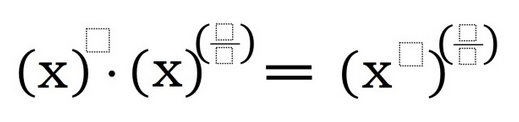# Rational Exponents 2

Directions: Using any number between 1 and 9, fill in the boxes to create a true statement. You may only use a number once.### Hint

What are the properties of exponents? How do they relate?

### Answer

There are many possible solutions, one is:
x^3*x^(1/2) = (x^7)^(4/8)

Source: Bryan Anderson

## Properties of Exponents 4

Directions: Using the digits 1 to 9 at most one time each, fill in the …